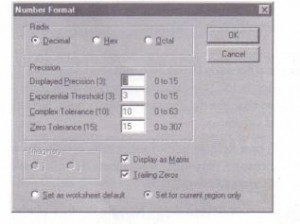The way that Mathcad displays numbers (the number of decimal places, whether to use i or j for imaginary numbers, and so on) is called the result format. You can set the result format for a whole worksheet or for a single calculated result. Setting worksheet default format To change the default display of numerical results, click on an empty space and choose Number from the Format menu. You will see the dialog box shown below. Default values for the four precision settings are shown in parentheses. The range of values allowed for each setting is shown to the right of the corresponding text box.Change the desired settings and click “OK.” Mathcad changes the display of all results whose formats have not been explicitly specified. You can control the display of a single result without affecting any others by setting the local result format. The section “Setting the format of a single result” on page 120 discusses how to do this. This is the meaning of each of the settings in the dialog box:

This allows you to set the radix (base) in which results are displayed. The default is decimal. If you select “Octal” or “Hex,” Mathcad truncates the number to an integer and displays it as octal or hexadecimal. Hexadecimal results are indicated by the letter “h” after the number, octal results by the letter “0.” (Mathcad does not reliably show hexadecimal or octal numbers of magnitude greater than 231 ,or about 2· 109).

Imaginary

Click on the i or j button. Mathcad uses the selected letter to indicate an imaginary result. The default is i. You can use either i or j in equations-Mathcad always understands both. The selection you make here tells Mathcad which one to use when it gives you a complex answer. See the section “Complex numbers” in Chapter 8

Display as Matrix

Check this box to suppress the use of scrolling output tables. Vectors and matrices having more than nine rows or columns are displayed as scrolling output tables unless this box is checked. See the section “Displaying vectors and matrices” in Chapter 10 for more information on scrolling output tables.

Trailing Zeros

Check this box to make all displayed results have as many digits to the right of the decimal point as required by the current choice of Displayed Precision. For example, with Displayed Precision set to 3, Mathcad displays 5 as 5.000.

Displayed Precision

Enter an integer between 0 and 15. This indicates how many decimal places to show in computed results. The default is 3. Numbers are rounded for display only. Mathcad maintains 15 digits of precision internally.

Exponential Threshold

Enter an integer n between 0 and 15. Mathcad shows computed results of magnitude greater than IOn or smaller than IO-n in exponential notation. When the threshold is 3, numbers like 30,000 are displayed as 3 . 104 instead of 30,000. The default is
3.

Complex Tolerance

Enter an integer n between 0 and 63. If the ratio between the real and imaginary part of a complex number is less than Ion or greater than lo-n, then the smaller part is not shown. The default setting is 10. This means that numbers like 1 + 1O-12j :Will appear simply as 1. Mathcad rounds only the displayed values. No change is made in the internally stored values.

Zero Tolerance

Enter an integer n between 0 and 307. Numbers less than IOn are shown as zero. The default setting is 15. This means numbers of magnitude less than 10-15 appear as zero. Mathcad changes the displayed values only. No change is made in the

internally stored values.

Changing the worksheet default result format affects only the worksheet you were in when you made the change. Any other worksheets that may have been open at the time retain their own default result formats. If you want to re-use your default result formats in other Mathcad worksheets, save your worksheet as a template as described in Chapter 4, “Worksheet Management.” All of these formatting options affect only the display of a number. Mathcad continues to carry out all calculations in full precision regardless of formatting options. If you copy a result by choosing Copy from the Edit menu, Mathcad copies the answer to the precision displayed. To see the number as it is stored internally:
• Click anywhere on the result.
• Press [Ctrl][Shift]N .

Mathcad displays the number in full precision on the message line at the bottom of  the application window, as shown in Figure 6-1.

Setting the format of a single result

You can set the format for a single result-a number, table, vector, or matrixindependently of the worksheet default result format. To change the format with which a particular result is displayed, do the following:
• Click anywhere in the equation whose result you want to format.
• Choose Number from the Format menu. Alternatively, double-click on the equation itself. The dialog box shown below appears. Note that the option button “Set for current region only” is already selected Setting the format of a single result You can set the format for a single result-a number, table, vector, or matrix independently
of the worksheet default result format. To change the format with which a particular result is displayed, do the following:
• Click anywhere in the equation whose result you want to format.
• Choose Number from the Format menu. Alternatively, double-click on the equation itself. The dialog box shown below appears. Note that the option button “Set for current region only” is already selected• Change the desired settings. See the section “Setting worksheet default format” on page 118 to learn what the various settings do and what their allowed values are. To display the six decimal places shown below, you would change “Displayed Precision” from 3 to 6.
• Click “OK.” Mathcad redisplays the result using the new forma

Posted on November 21, 2015 in Equation and Result Formatting